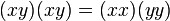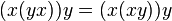# Square map is endomorphism iff abelian

This article describes an easy-to-prove fact about basic notions in group theory, that is not very well-known or important in itself
View other elementary non-basic facts
VIEW FACTS USING THIS: directly | directly or indirectly, upto two steps | directly or indirectly, upto three steps|

## Statement

Let$G$ be a group and$\sigma:G \to G$ be the square map of$G$ defined as$\sigma(x) = x^2$. Then,$\sigma$ is an endomorphism of$G$ (i.e.,$\sigma(xy) = \sigma(x)\sigma(y) \ \forall \ x,y \in G$) if and only if$G$ is abelian.

Another way of putting it is that$G$ is 2-abelian if and only if it is abelian.

## Related facts

### Other$n^{th}$ power maps

The$n^{th}$ power map for a fixed integer$n$ is termed a universal power map, and if it is also an endomorphism, it is termed a universal power endomorphism and the group is termed a n-abelian group. This statement gives a necessary and sufficient condition for a group where$n = 2$ gives an endomorphism. Here are results for other values of$n$.

Value of$n$ (note that the condition for$n$ is the same as the condition for$1-n$) Characterization of$n$-abelian groups Proof Other related facts
0 all groups obvious
1 all groups obvious
2 abelian groups only 2-abelian iff abelian endomorphism sends more than three-fourths of elements to squares implies abelian
-1 abelian groups only -1-abelian iff abelian
3 2-Engel group and derived subgroup has exponent dividing three Levi's characterization of 3-abelian groups cube map is surjective endomorphism implies abelian, cube map is endomorphism iff abelian (if order is not a multiple of 3)
-2 same as for 3-abelian (based on n-abelian iff (1-n)-abelian)

### Related facts for Lie rings

Here are some related facts for Lie rings:

### Opposite facts for other algebraic structures

Statement Algebraic structure What step of the proof fails? Comment
Square map is endomorphism not implies abelian for loop loop The reparenthesization in Step (3) of the proof below, that requires associativity. In fact, it is possible to have a noncommutative loop of exponent two.
Square map is endomorphism not implies abelian for monoid monoid The cancellation in Step (4), which requires that we are working over a cancellative monoid.

## Facts used

1. Associative implies generalized associative: Basically this says that in a group, we can drop and rearrange parentheses at will.
2. Invertible implies cancellative in monoid. Since every element of a group is invertible, cancellation is valid in groups.
3. Abelian implies universal power map is endomorphism

## Proof

### From square map being endomorphism to abelian

This proof uses a tabular format for presentation. Provide feedback on tabular proof formats in a survey (opens in new window/tab) | Learn more about tabular proof formats|View all pages on facts with proofs in tabular format

Given: A group$G$ such that the map$\sigma = x \mapsto x^2$ is an endomorphism, i.e.,$(xy)^2 = x^2y^2$ for all$x,y \in G$.

To prove:$xy = yx$ for all$x,y \in G$.

Proof: We let$x,y$ be arbitrary elements of$G$.

Step no. Assertion/construction Facts used Given data used Previous steps used Explanation What algebraic assumptions does this use?
1$(xy)^2 = (x^2)(y^2)$ -- square map is endomorphism -- -- None, works over any magma
2$(xy)(xy) = (xx)(yy)$ -- Step (1) -- None, just using definition of square. Works over any magma.
3$(x(yx))y = (x(xy))y$ Fact (1) Step (2) Reparenthesize The reparenthesization requires associativity of expressions involving two variables. It works over any semigroup or monoid and even more generally over any diassociative magma.
4$yx = xy$ Fact (2) Step (3) Cancel the right-most$y$ from both sides, then the left-most$x$ from both sides. The cancellation requires that we are working in a cancellative magma, such as a cancellative monoid or a quasigroup or loop.

### From abelian to square map being endomorphism

This follows directly from fact (3).